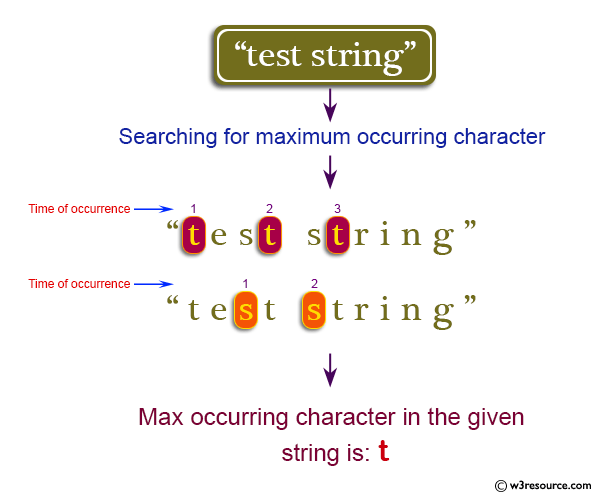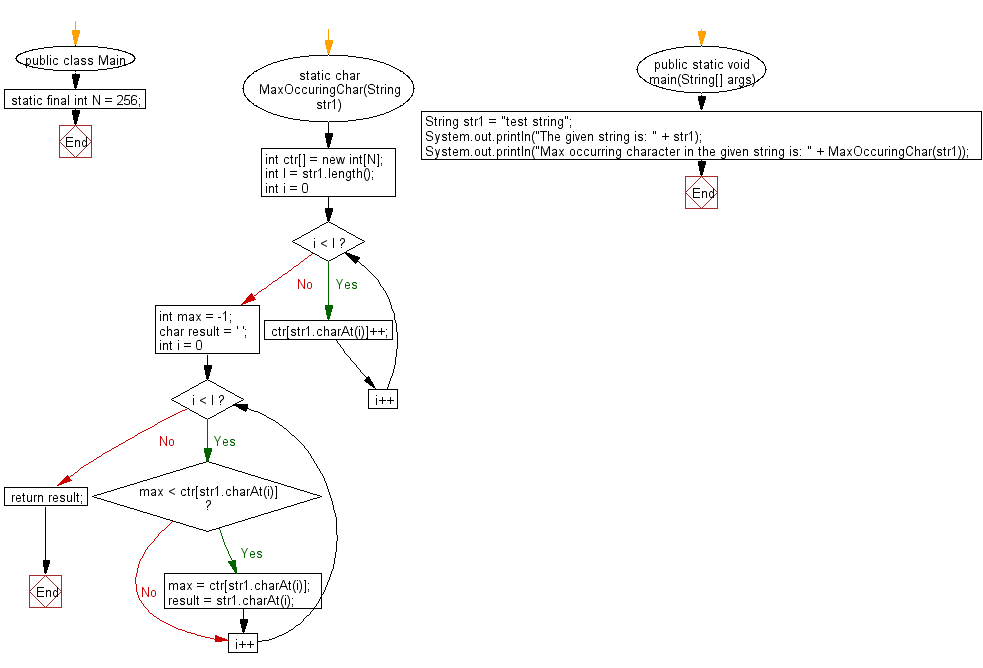﻿ Java exercises: Find the maximum occurring character in a string - w3resource# Java String Exercises: Find the maximum occurring character in a string

## Java String: Exercise-43 with Solution

Write a Java program to find the maximum occurring character in a string.

Pictorial Presentation:Sample Solution:

Java Code:

``````import java.util.*;
public class Main {
static final int N = 256;
static char MaxOccuringChar(String str1) {
int ctr[] = new int[N];
int l = str1.length();
for (int i = 0; i < l; i++)
ctr[str1.charAt(i)]++;
int max = -1;
char result = ' ';

for (int i = 0; i < l; i++) {
if (max < ctr[str1.charAt(i)]) {
max = ctr[str1.charAt(i)];
result = str1.charAt(i);
}
}

return result;
}
public static void main(String[] args) {
String str1 = "test string";
System.out.println("The given string is: " + str1);
System.out.println("Max occurring character in the given string is: " + MaxOccuringChar(str1));
}
}
```
```

Sample Output:

```The given string is: test string
Max occurring character in the given string is: t
```

Flowchart:Java Code Editor:

Improve this sample solution and post your code through Disqus

What is the difficulty level of this exercise?

﻿

New Content: Composer: Dependency manager for PHP, R Programming